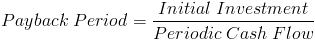Custom Search

# Payback Period

Payback Period Calculator (Click Here or Scroll Down)The payback period formula is used to determine the length of time it will take to recoup the initial amount invested on a project or investment. The payback period formula is used for quick calculations and is generally not considered an end-all for evaluating whether to invest in a particular situation.

The result of the payback period formula will match how often the cash flows are received. An example would be an initial outflow of \$5,000 with \$1,000 cash inflows per month. This would result in a 5 month payback period. If the cash inflows were paid annually, then the result would be 5 years.

At times, the cash flows will not be equal to one another. If \$10,000 is the initial investment and the cash flows are \$1,000 at year one, \$6,000 at year two, \$3,000 at year three, and \$5,000 at year four, the payback period would be three years as the first three years are equal to the initial outflow.

### Use of Payback Period Formula

There are a few drawbacks to the payback period formula that may warrant one to consider using another method of determining whether to invest.

One issue is that the payback period formula does not look at the value of all returns. Suppose a situation where there are two choices to choose from where investment X has a payback period of 1 year and investment Y has a payback period of 2 years. However, investment X will only return the initial investment whereas investment Y will eventually pay double the initial investment. Given the additional information not provided by the payback period formula, one may consider investment Y to be preferable. The formula for the net present value method may be used to close this information gap in order to properly evaluate the best choice.

However, it is worth mentioning that although the net present value method may be preferable to determine long term profitability, the payback period formula helps with cash flow analysis for short term budgeting. Suppose a situation where investment X has a net present value of 10% more than its initial investment and investment Y has a net present value of triple its initial investment. At first glance, investment Y may seem the reasonable choice, but suppose that the payback period for investment X is 1 year and investment Y is 10 years. Investment Y could cause problems if the investment is needed sooner. An analogy of this would be like banks where maintaining cash flows of their investments(loans) is vital to their business.

Another issue with the formula for period payback is that it does not factor in the time value of money. The time value of money concept, as it applies to the payback period formula, proposes that each future cash flow is worth less when compared to today's value. The discounted payback period formula may be used instead to consider the time value of money, however the discounted payback period formula takes away the benefit of making quick calculations.

New to Finance?